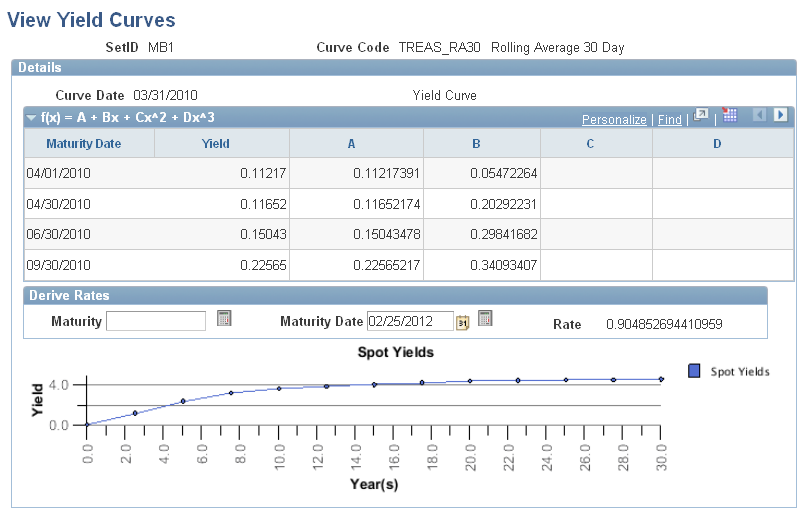Viewing Yield Curves

Yield curves are constructed and stored in the database as ranges of maturities for which a single cubic equation (y = a + bx + cx2 + dx3) describes the yield curve through those maturities. A complete yield curve for maturities from zero through thirty years or longer is typically made up of a few of these equations, each describing a segment of the curve. You can use the equations making up the complete curve in a spreadsheet for graphing purposes. Individual forward rates can be calculated online by using this page.

Page Used to View Generated Yield Curves

Page Name

Definition Name

Usage

View Yield Curves

YCEQS_PNL

select Financial Services Industries, then select Interest Rate Environment, then select Yield Curve Inquiry, then select View Yield Curves

View constructed curves online. The cubic equations that make up each page can be viewed once Curve Generator constructs a yield curve (that is, once you've run the yield curve jobstream).

View Yield Curves Page

Use the View Yield Curves page (YCEQS_PNL) to view constructed curves online.

The cubic equations that make up each page can be viewed once Curve Generator constructs a yield curve (that is, once you've run the yield curve jobstream).

Image: View Yield Curves page

This example illustrates the fields and controls on the View Yield Curves page. You can find definitions for the fields and controls later on this page.To view yield curves, enter a value in the Maturity field. Enter a data in theMaturity Dt field. Today's date is the default. Click theCalculate button next to theMaturity field. The rate appears in theRate field.